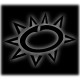# motor

The selected motor is the D2830-11 has the following  specifications:

• power supply:7,5 -15 V
• Kv: 1000 RPM/V
• Max Power: 210 W
• suggested: ESC 30A, battery Lipox2 with props 10×4.7 or battery Lipox4 with props 8×4

1. The rotational speed is only related to the supplied voltage.
2. Considering I’m using a battery Lipox3 – 11.1V,  the motor can reach a speed of RPMmax=Kv*V=11100 rpm = 185 rps
3. The Max current is  A=W/V=18.9 A ,around 20 A
4. Power can be approximated by:  W=Kp*D^4*P*RPM^3. Where Kp is a constant related to the prop,D is the prop diameter, P is the pitch of the prop.
5. If yuo conenct the 2 preview formulas   A=Kp*D^4*P*RPM^3/V=Kp*D^4*P*Kv^3*V^2.  The current can increase withthe cube of kv and with square of V.

It is possible to extimate all the quadcopter parameter using this  ecalc  . Here the calculation related to my hardware.

Considering that it is suggested to keep the Throttle % < 80% , it means that quadcopter  weight has to be less than 1,5 Kg

## 4 thoughts on “motor”

1.okierie

this blog is a good reference. i will need it soon! 😀

2.okierie

Can you explain why do we need CW and CCW motors? What is the point of using CW and CCW motors (together)? Can we just use CW or CCW only?

1.solenerotech Post author

Hi okierie
The rotation of one prop generate a torque.
So if you have 2 props rotating in opposite ways the resultant of the torque is null.In this way the drone does not rotate along its z axis.
Take a look on this theory introduction: https://solenerotech1.wordpress.com/quadcopter/